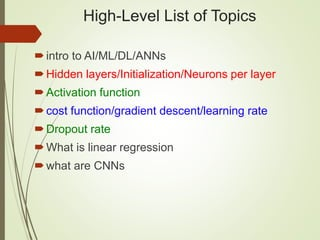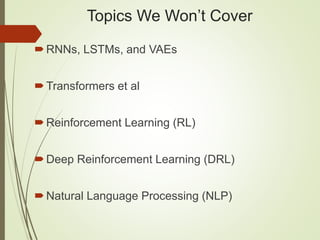Successfully reported this slideshow.

# Introduction to Deep Learning×

# Introduction to Deep Learning

A fast-paced introduction to Deep Learning concepts, such as activation functions, cost functions, back propagation, and then a quick dive into CNNs. Basic knowledge of vectors, matrices, and derivatives is helpful in order to derive the maximum benefit from this session.

A fast-paced introduction to Deep Learning concepts, such as activation functions, cost functions, back propagation, and then a quick dive into CNNs. Basic knowledge of vectors, matrices, and derivatives is helpful in order to derive the maximum benefit from this session.

### Introduction to Deep Learning

1. 1. Introduction to Deep Learning UCSC Meetup (Santa Clara) Monday 08/12/2019 Oswald Campesato ocampesato@yahoo.com
2. 2. High-Level List of Topics intro to AI/ML/DL/ANNs Hidden layers/Initialization/Neurons per layer Activation function cost function/gradient descent/learning rate Dropout rate What is linear regression what are CNNs
3. 3. Topics We Won’t Cover RNNs, LSTMs, and VAEs Transformers et al Reinforcement Learning (RL) Deep Reinforcement Learning (DRL) Natural Language Processing (NLP)
4. 4. The Data/AI Landscape
5. 5. The Official Start of AI (1956)
6. 6. Use Cases for Deep Learning computer vision speech recognition image processing bioinformatics social network filtering drug design Customer relationship management Recommendation systems Bioinformatics Mobile Advertising Many others
7. 7. What is a Deep Neural Network?  input layer, multiple hidden layers, and output layer  nonlinear processing via activation functions  perform transformation and feature extraction  gradient descent algorithm with back propagation  each layer receives the output from previous layer  results are comparable/superior to human experts
8. 8. Types of Deep Learning  Supervised learning (you know the answer)  unsupervised learning (you don’t know the answer)  Semi-supervised learning (mixed dataset)  Reinforcement learning (such as games)  Types of algorithms:  Classifiers (detect images, spam, fraud, etc)  Regression (predict stock price, housing price, etc)  Clustering (unsupervised classifiers)
9. 9. Deep Learning Architectures Unsupervised Pre-trained Networks (UPNs) Convolutional Neural Networks (CNNs) Recurrent Neural Networks (LSTMs) The most popular architectures: CNNs (and capsule networks) for images LSTMs for NLP and audio
10. 10. Neural Network: 3 Hidden Layers
11. 11. NN: 2 Hidden Layers (Regression)
12. 12. Titanic Dataset (portion)
13. 13. Classification and Deep Learning
14. 14. What is Linear Regression One of the simplest models in ML Fits a line (y = m*x + b) to data in 2D Finds best line by minimizing MSE: m: = minimize sum of squared values b also has a closed form solution
15. 15. Linear Regression in 2D: example
16. 16. Linear Regression in 2D: example
17. 17. Sample Cost Function #1 (MSE)
18. 18. Linear Regression: example #1 One feature (independent variable): X = number of square feet Predicted value (dependent variable): Y = cost of a house A very “coarse grained” model We can devise a much better model
19. 19. Linear Regression: example #2 Multiple features: X1 = # of square feet X2 = # of bedrooms X3 = # of bathrooms (dependency?) X4 = age of house X5 = cost of nearby houses X6 = corner lot (or not): Boolean a much better model (6 features)
20. 20. Linear Multivariate Analysis General form of multivariate equation: Y = w1*x1 + w2*x2 + . . . + wn*xn + b w1, w2, . . . , wn are numeric values x1, x2, . . . , xn are variables (features) Properties of variables: Can be independent (Naïve Bayes) weak/strong dependencies can exist
21. 21. Neural Network with 3 Hidden Layers
22. 22. Neural Networks (general) Multiple hidden layers: Layer composition is your decision Activation functions: sigmoid, tanh, RELU https://en.wikipedia.org/wiki/Activation_function Back propagation (1980s) https://en.wikipedia.org/wiki/Backpropagation => Initial weights: small random numbers
23. 23. Euler’s Function (e: 2.71828. . .)
24. 24. The sigmoid Activation Function
25. 25. The tanh Activation Function
26. 26. The ReLU Activation Function
27. 27. The softmax Activation Function
28. 28. Gaussian Functions
29. 29. Gaussian Functions
30. 30. Activation Functions in Python import numpy as np ... # Python sigmoid example: z = 1/(1 + np.exp(-np.dot(W, x))) ... # Python tanh example: z = np.tanh(np.dot(W,x)); # Python ReLU example: z = np.maximum(0, np.dot(W, x))
31. 31. What’s the “Best” Activation Function? Initially: sigmoid was popular Then: tanh became popular Now: RELU is preferred (better results) Softmax: for FC (fully connected) layers NB: sigmoid and tanh are used in LSTMs
32. 32. Types of Cost/Error Functions MSE (mean-squared error) Cross-entropy others
33. 33. Sample Cost Function #1 (MSE)
34. 34. Sample Cost Function #2
35. 35. Sample Cost Function #3
36. 36. How to Select a Cost Function mean-squared error: for a regression problem binary cross-entropy (or mse): for a two-class classification problem categorical cross-entropy: for a many-class classification problem
38. 38. Setting up Data & the Model standardize the data: Subtract the ‘mean’ and divide by stddev Initial weight values for NNs: random(0,1) or N(0,1) or N(0/(1/n)) More details: http://cs231n.github.io/neural-networks-2/#losses
39. 39. Hyper Parameters (examples) # of hidden layers in a neural network the learning rate (in many models) the dropout rate # of leaves or depth of a tree # of latent factors in a matrix factorization # of clusters in a k-means clustering
40. 40. Hyper Parameter: dropout rate "dropout" refers to dropping out units (both hidden and visible) in a neural network a regularization technique for reducing overfitting in neural networks prevents complex co-adaptations on training data a very efficient way of performing model averaging with neural networks
41. 41. How Many Layers in a DNN? Algorithm #1 (from Geoffrey Hinton): 1) add layers until you start overfitting your training set 2) now add dropout or some another regularization method Algorithm #2 (Yoshua Bengio): "Add layers until the test error does not improve anymore.”
42. 42. How Many Hidden Nodes in a DNN? Based on a relationship between: # of input and # of output nodes Amount of training data available Complexity of the cost function The training algorithm TF playground home page: http://playground.tensorflow.org
43. 43. CNNs versus RNNs CNNs (Convolutional NNs): Good for image processing 2000: CNNs processed 10-20% of all checks => Approximately 60% of all NNs RNNs (Recurrent NNs): Good for NLP and audio Used in hybrid networks
44. 44. CNNs: Convolution, ReLU, and Max Pooling
45. 45. CNNs: Convolution Calculations https://docs.gimp.org/en/plug-in-convmatrix.html
46. 46. CNNs: Convolution Matrices (examples) Sharpen: Blur:
47. 47. CNNs: Convolution Matrices (examples) Edge detect: Emboss:
48. 48. CNNs: Convolution Matrices (examples)
49. 49. CNNs: Convolution Matrices (examples)
50. 50. CNNs: Convolution Matrices (examples)
51. 51. CNN Filter Terminology Stride: how many units you “shift” the filter (1/2/3/etc) Padding: makes feature map same size as image Kernel size: the dimensions of the filter (1x1, 3x3, 5x5, etc)
52. 52. CNNs: Max Pooling Example
53. 53. Components of a CNN (again) 1) Specify the input layer 2) Add a convolution to create feature maps 3) Perform RELU on the feature maps 4) repeat 1) and 2) 5) add a FC (fully connected layer) 6) connect FC to output layer via softmax
54. 54. CNN pseudocode  Specify an optimiser  specify a cost function  specify a learning rate  Specify desired metrics (accuracy/precision/etc)  specify # of batch runs in a training epoch  For each epoch:  For each batch:  Extract the batch data  Run the optimiser + cross-entropy operations  Add to the average cost  Calculate the current test accuracy  Print out some results  Calculate the final test accuracy and print
55. 55. CNN in Python/Keras (fragment)  from keras.models import Sequential  from keras.layers.core import Dense, Dropout, Flatten, Activation  from keras.layers.convolutional import Conv2D, MaxPooling2D  from keras.optimizers import Adadelta  input_shape = (3, 32, 32)  nb_classes = 10  model = Sequential()  model.add(Conv2D(32, (3, 3), padding='same’, input_shape=input_shape))  model.add(Activation('relu'))  model.add(Conv2D(32, (3, 3)))  model.add(Activation('relu'))  model.add(MaxPooling2D(pool_size=(2, 2)))  model.add(Dropout(0.25))
56. 56. GANs: Generative Adversarial Networks
57. 57. GANs: Generative Adversarial Networks Make imperceptible changes to images Can consistently defeat all NNs Can have extremely high error rate Some images create optical illusions https://www.quora.com/What-are-the-pros-and-cons- of-using-generative-adversarial-networks-a-type-of- neural-network